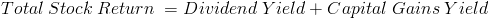# Total Stock ReturnThe formula for the total stock return is the appreciation in the price plus any dividends paid, divided by the original price of the stock. The income sources from a stock is dividends and its increase in value. The first portion of the numerator of the total stock return formula looks at how much the value has increased (P1 - P0). The denominator of the formula to calculate a stock's total return is the original price of the stock which is used due to being the original amount invested.

## Total Stock Return Cash Amount

The formula shown at the top of the page is used to calculate the percentage return. The actual cash amount for the total stock return can be calculated using only the numerator of the percentage return formula.For example, assume that an individual originally paid \$1000 for a particular stock that has paid dividends of \$20 and the ending price is \$1020. The total return would be \$40 which equals \$1020 minus \$1000, then plus \$20.

## Example of the Total Stock Return Formula

Using the prior example, the original price is \$1000 and the ending price is \$1020. The appreciation of the stock is then \$20. The \$20 in price appreciation can then be added to dividends of \$20 which would equal a total return of \$40. This can then be divided by the original price of \$1000 which would equal a percentage return of 4%.

## Alternative Total Stock Return FormulaThe total stock return can also be calculated by adding the dividend yield to the capital gains yield. The capital gains yield may sometimes be shown as the percentage change in stock price. This alternative formula is derived from separating the stock appreciation and dividends in the formula shown at the top of the page which becomes the capital gains yield and the dividend yield.

New to Finance?